Home
Hostname: page-component-544b6db54f-lmg95 Total loading time: 0.593 Render date: 2021-10-22T13:34:48.558Z Has data issue: true Feature Flags: { "shouldUseShareProductTool": true, "shouldUseHypothesis": true, "isUnsiloEnabled": true, "metricsAbstractViews": false, "figures": true, "newCiteModal": false, "newCitedByModal": true, "newEcommerce": true, "newUsageEvents": true }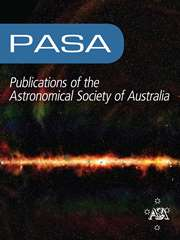Publications of the Astronomical Society of Australia

## Abstract

Recent X-ray observations by Jiang et al. have identified an active galactic nucleus (AGN) in the bulgeless spiral galaxy NGC 3319, located just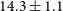$14.3\pm 1.1$ Mpc away, and suggest the presence of an intermediate-mass black hole (IMBH;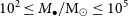$10^2\leq M_\bullet/\textrm{M}_{\odot}\leq 10^5$ ) if the Eddington ratios are as high as 3 to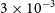$3\times10^{-3}$ . In an effort to refine the black hole mass for this (currently) rare class of object, we have explored multiple black hole mass scaling relations, such as those involving the (not previously used) velocity dispersion, logarithmic spiral arm pitch angle, total galaxy stellar mass, nuclear star cluster mass, rotational velocity, and colour of NGC 3319, to obtain 10 mass estimates, of differing accuracy. We have calculated a mass of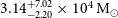$3.14_{-2.20}^{+7.02}\times10^4\,\textrm{M}_\odot$ , with a confidence of 84% that it is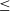$\leq$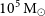$10^5\,\textrm{M}_\odot$ , based on the combined probability density function from seven of these individual estimates. Our conservative approach excluded two black hole mass estimates (via the nuclear star cluster mass and the fundamental plane of black hole activity—which only applies to black holes with low accretion rates) that were upper limits of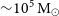${\sim}10^5\,{\textrm M}_{\odot}$ , and it did not use the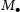$M_\bullet$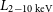$L_{\textrm 2-10\,\textrm{keV}}$ relation’s prediction of$\sim$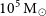$10^5\,{\textrm M}_{\odot}$ . This target provides an exceptional opportunity to study an IMBH in AGN mode and advance our demographic knowledge of black holes. Furthermore, we introduce our novel method of meta-analysis as a beneficial technique for identifying new IMBH candidates by quantifying the probability that a galaxy possesses an IMBH.

Type
Research Article
© The Author(s), 2021. Published by Cambridge University Press on behalf of the Astronomical Society of Australia

## Access options

Get access to the full version of this content by using one of the access options below. (Log in options will check for institutional or personal access. Content may require purchase if you do not have access.)

## References

Abbott, B. P., et al. 2019a, PhRvX, 9, 031040 Google Scholar
Abbott, B. P., et al. 2019b, PhRvD, 100, 064064 Google Scholar
Abbott, R., et al. 2020a, arXiv e-prints, p. arXiv:2010.14527 Google Scholar
Abbott, R., et al. 2020b, PhRvL, 125, 101102 Google Scholar
Abbott, R., et al. 2020c, ApJ, 896, L44 CrossRefGoogle Scholar
Abbott, R., et al. 2020d, ApJ, 900, L13 CrossRefGoogle Scholar
Abdeen, S., Kennefick, D., Kennefick, J., Miller, R., Shields, D. W., Monson, E. B., & Davis, B. L. 2020, MNRAS, 496, 1610 CrossRefGoogle Scholar
Abramowicz, M. A., Calvani, M., & Nobili, L. 1980, ApJ, 242, 772 CrossRefGoogle Scholar
Akritas, M. G., & Bershady, M. A. 1996, ApJ, 470, 706 CrossRefGoogle Scholar
Alam, S., et al. 2015, ApJS, 219, 12 CrossRefGoogle Scholar
Alexander, T., & Natarajan, P. 2014, Sci, 345, 1330 CrossRefGoogle Scholar
Alister Seguel, P. J., Schleicher, D. R. G., Boekholt, T. C. N., Fellhauer, M., & Klessen, R. S. 2020, MNRAS, 493, 2352 CrossRefGoogle Scholar
Aller, M. C., & Richstone, D. 2002, AJ, 124, 3035 CrossRefGoogle Scholar
Amaro-Seoane, P., et al. 2017, arXiv e-prints, p. arXiv:1702.00786 Google Scholar
An, T., Sohn, B. W., & Imai, H. 2018, NatAs, 2, 118 Google Scholar
Andreoni, I., Jacobs, C., Hegarty, S., Pritchard, T., Cooke, J., & Ryder, S. 2017, PASA, 34, e037 CrossRefGoogle Scholar
Andreoni, I., et al. 2020, MNRAS, 491, 5852 CrossRefGoogle Scholar
Anninos, P., Hoffman, R. D., Grewal, M., Lavell, M. J., & Fragile, P. C. 2019, ApJ, 885, 136 CrossRefGoogle Scholar
Arca-Sedda, M., Amaro-Seoane, P., & Chen, X. 2020, arXiv e-prints, p. arXiv:2007.13746 Google Scholar
Baldassare, V. F., Dickey, C., Geha, M., & Reines, A. E. 2020, ApJ, 898, L3 CrossRefGoogle Scholar
Baldassare, V. F., Reines, A. E., Gallo, E., & Greene, J. E. 2015, ApJ, 809, L14 CrossRefGoogle Scholar
Baldassare, V. F., Reines, A. E., Gallo, E., & Greene, J. E. 2017, ApJ, 850, 196 CrossRefGoogle Scholar
Baldi, R. D., et al. 2018, MNRAS, 476, 3478 CrossRefGoogle Scholar
Baldwin, J. A., Phillips, M. M., & Terlevich, R. 1981, PASP, 93, 5 CrossRefGoogle Scholar
Barrows, R. S., Mezcua, M., & Comerford, J. M. 2019, ApJ, 882, 181 CrossRefGoogle Scholar
Belczynski, K., Bulik, T., Fryer, C. L., Ruiter, A., Valsecchi, F., Vink, J. S., & Hurley, J. R. 2010, ApJ, 714, 1217 CrossRefGoogle Scholar
Bellovary, J. M., et al. 2021, arXiv e-prints, p. arXiv:2102.09566 Google Scholar
Bentz, M. C., & Katz, S. 2015, PASP, 127, 67 CrossRefGoogle Scholar
Berrier, J. C., et al. 2013, ApJ, 769, 132 CrossRefGoogle Scholar
Bi, S., Feng, H., & Ho, L. C. 2020, ApJ, 900, 124 CrossRefGoogle Scholar
Bouquin, A. Y. K., et al. 2018, ApJS, 234, 18 CrossRefGoogle Scholar
Bromm, V., & Loeb, A. 2003, in American Institute of Physics Conference Series, Vol. 666, The Emergence of Cosmic Structure, ed. Holt, S. H., & Reynolds, C. S., 73 (arXiv:astro-ph/0301406), 10.1063/1.1581773 Google Scholar
Brum, C., et al. 2019, MNRAS, 486, 691 CrossRefGoogle Scholar
Burke, C. J., Shen, Y., Chen, Y.-C., Scaringi, S., Faucher-Giguere, C.-A., Liu, X., & Yang, Q. 2020, ApJ, 899, 136 CrossRefGoogle Scholar
Cappellari, M. 2017, MNRAS, 466, 798 CrossRefGoogle Scholar
Chilingarian, I. V., Katkov, I. Y., Zolotukhin, I. Y., Grishin, K. A., Beletsky, Y., Boutsia, K., & Osip, D. J. 2018, ApJ, 863, 1 CrossRefGoogle Scholar
Cho, H., et al. 2020, ApJ, 892, 93 CrossRefGoogle Scholar
Ciambur, B. C. 2015, ApJ, 810, 120 CrossRefGoogle Scholar
Ciambur, B. C. 2016, PASA, 33, e062 CrossRefGoogle Scholar
Ciotti, L. 1991, A&A, 249, 99 Google Scholar
Combes, F., et al. 2019, A&A, 623, A79 Google Scholar
Croton, D. J., et al. 2006, MNRAS, 365, 11 CrossRefGoogle Scholar
Davies, M. B., Miller, M. C., & Bellovary, J. M. 2011, ApJ, 740, L42 CrossRefGoogle Scholar
Davis, B. L., Berrier, J. C., Shields, D. W., Kennefick, J., Kennefick, D., Seigar, M. S., Lacy, C. H. S., & Puerari, I. 2012, ApJS, 199, 33 CrossRefGoogle Scholar
Davis, B. L., et al. 2014, ApJ, 789, 124 CrossRefGoogle Scholar
Davis, B. L., et al. 2015, ApJ, 802, L13 CrossRefGoogle Scholar
Davis, B. L., Berrier, J. C., Shields, D. W., Kennefick, J., Kennefick, D., Seigar, M. S., Lacy, C. H. S., & Puerari, I. 2016, 2DFFT: Measuring Galactic Spiral Arm Pitch Angle (ascl:1608.015)Google Scholar
Davis, B. L., Graham, A. W., & Seigar, M. S. 2017, MNRAS, 471, 2187 CrossRefGoogle Scholar
Davis, B. L., Graham, A. W., & Cameron, E. 2018, ApJ, 869, 113 CrossRefGoogle Scholar
Davis, B. L., Graham, A. W., & Cameron, E. 2019a, ApJ, 873, 85 CrossRefGoogle Scholar
Davis, B. L., Graham, A. W., & Combes, F. 2019b, ApJ, 877, 64 CrossRefGoogle Scholar
Davis, D. R., & Hayes, W. B. 2014, ApJ, 790, 87 CrossRefGoogle Scholar
Davis, T. A., et al. 2020, MNRAS, 496, 4061 CrossRefGoogle Scholar
den Brok, M., et al. 2015, ApJ, 809, 101 CrossRefGoogle Scholar
de Vaucouleurs, G., de Vaucouleurs, A., Corwin Herold, G. J., Buta, R. J., Paturel, G., & Fouque, P. 1991, Third Reference Catalogue of Bright Galaxies (New York: Springer)CrossRefGoogle Scholar
Dong, A.-J., & Wu, Q. 2015, MNRAS, 453, 3447 CrossRefGoogle Scholar
D’Onghia, E., Vogelsberger, M., & Hernquist, L. 2013, ApJ, 766, 34 CrossRefGoogle Scholar
Driver, S. P., Popescu, C. C., Tuffs, R. J., Graham, A. W., Liske, J., & Baldry, I. 2008, ApJ, 678, L101 CrossRefGoogle Scholar
Dullo, B. T., Bouquin, A. Y. K., Gil de Paz, A., Knapen, J. H., & Gorgas, J. 2020, ApJ, 898, 83 CrossRefGoogle Scholar
Eddington, A. S. 1925, MNRAS, 85, 408 CrossRefGoogle Scholar
Edri, H., Rafter, S. E., Chelouche, D., Kaspi, S., & Behar, E. 2012, ApJ, 756, 73 CrossRefGoogle Scholar
Edwards, P. G., & Phillips, C. 2015, PKoAS, 30, 659 Google Scholar
Elvis, M., et al. 1994, ApJS, 95, 1 CrossRefGoogle Scholar
Event Horizon Telescope Collaboration, et al. 2019, ApJ, 875, L1 CrossRefGoogle Scholar
Falcke, H., Körding, E., & Markoff, S. 2004, A&A, 414, 895 Google Scholar
Farrell, S. A., Webb, N. A., Barret, D., Godet, O., & Rodrigues, J. M. 2009, Nat, 460, 73 CrossRefGoogle Scholar
Feng, H., & Soria, R. 2011, NewAR, 55, 166 CrossRefGoogle Scholar
Ferrers, N. 1877, QJ PAM, 14, 1 Google Scholar
Fonseca, E., et al. 2021, arXiv e-prints, p. arXiv:2104.00880 Google Scholar
Fragione, G., & Silk, J. 2020, MNRAS, 498, 4591 CrossRefGoogle Scholar
García-Burillo, S., et al. 2014, A&A, 567, A125 Google Scholar
Georgiev, I. Y., & Böker, T. 2014, MNRAS, 441, 3570 CrossRefGoogle Scholar
Georgiev, I. Y., Böker, T., Leigh, N., Lützgendorf, N., & Neumayer, N. 2016, MNRAS, 457, 2122 CrossRefGoogle Scholar
Graham, A. W. 2012, ApJ, 746, 113 CrossRefGoogle Scholar
Graham, A. W. 2016a, in IAU Symposium, Vol. 312, Star Clusters and Black Holes in Galaxies across Cosmic Time, ed. Meiron, Y., Li, S., Liu, F. K., & Spurzem, R., 269 (arXiv:1412.5715), 10.1017/S1743921315008017 Google Scholar
Graham, A. W. 2016b, Galaxy Bulges and Their Massive Black Holes: A Review (Switzerland: Springer International Publishing), 263, 10.1007/978-3-319-19378-6_11 CrossRefGoogle Scholar
Graham, A. W. 2020, MNRAS, 492, 3263 CrossRefGoogle Scholar
Graham, A. W., Ciambur, B. C., & Soria, R. 2016, ApJ, 818, 172 CrossRefGoogle Scholar
Graham, A. W., & Driver, S. P. 2005, PASA, 22, 118 CrossRefGoogle Scholar
Graham, A. W., Driver, S. P., Allen, P. D., & Liske, J. 2007, MNRAS, 378, 198 CrossRefGoogle Scholar
Graham, A. W., Onken, C. A., Athanassoula, E., & Combes, F. 2011, MNRAS, 412, 2211 CrossRefGoogle Scholar
Graham, A. W., & Scott, N. 2013, ApJ, 764, 151 CrossRefGoogle Scholar
Graham, A. W., & Scott, N. 2015, ApJ, 798, 54 CrossRefGoogle Scholar
Graham, A. W., & Soria, R. 2019, MNRAS, 484, 794 Google Scholar
Graham, A. W., Soria, R., & Davis, B. L. 2019, MNRAS, 484, 814 Google Scholar
Graham, A. W., & Spitler, L. R. 2009, MNRAS, 397, 2148 CrossRefGoogle Scholar
Griv, E., Gedalin, M., Shih, I. C., Hou, L.-G., & Jiang, I.-G. 2020, MNRAS, 493, 2111 CrossRefGoogle Scholar
Griv, E., Gedalin, M., & Jiang, I.-G. 2021, MNRAS, 503, 354 CrossRefGoogle Scholar
Grobov, A. V., Rubin, S. G., Samarchenko, D. A., & Zhizhin, E. D. 2011, GC, 17, 181 Google Scholar
Gültekin, K., Cackett, E. M., King, A. L., Miller, J. M., & Pinkney, J. 2014, ApJ, 788, L22 CrossRefGoogle Scholar
Gültekin, K., Cackett, E. M., Miller, J. M., Di Matteo, T., Markoff, S., & Richstone, D. O. 2009, ApJ, 706, 404 CrossRefGoogle Scholar
Gültekin, K., King, A. L., Cackett, E. M., Nyland, K., Miller, J. M., Di Matteo, T., Markoff, S., & Rupen, M. P., 2019, ApJ, 871, 80 CrossRefGoogle Scholar
Haas, R., Shcherbakov, R. V., Bode, T., & Laguna, P. 2012, ApJ, 749, 117 CrossRefGoogle Scholar
Heckman, T. M. 1980, A&A, 500, 187 Google Scholar
Heckman, T. M., Balick, B., & Crane, P. C. 1980, A&AS, 40, 295 Google Scholar
Ho, L. C., Filippenko, A. V., & Sargent, W. L. 1995, ApJS, 98, 477 CrossRefGoogle Scholar
Ho, L. C., Filippenko, A. V., & Sargent, W. L. W. 1997, ApJS, 112, 315 CrossRefGoogle Scholar
Ho, L. C., Greene, J. E., Filippenko, A. V., & Sargent, W. L. W. 2009, ApJS, 183, 1 CrossRefGoogle Scholar
Inayoshi, K., Haiman, Z., & Ostriker, J. P. 2016, MNRAS, 459, 3738 CrossRefGoogle Scholar
Inayoshi, K., Visbal, E., & Haiman, Z. 2020, ARA&A, 58, 27 CrossRefGoogle Scholar
Jani, K., Shoemaker, D., & Cutler, C. 2019, NatAs, 4, 260 Google Scholar
Jayasinghe, T., et al. 2021, MNRAS, 504, 2577 CrossRefGoogle Scholar
Jiang, N., et al. 2018, ApJ, 869, 49 CrossRefGoogle Scholar
Jiang, Y.-F., Stone, J. M., & Davis, S. W. 2014, ApJ, 796, 106 CrossRefGoogle Scholar
Joye, W. A., & Mandel, E. 2003, in Astronomical Society of the Pacific Conference Series, Vol. 295, Astronomical Data Analysis Software and Systems XII, ed. H. E. Payne, R. I. Jedrzejewski, & R. N. Hook, 489Google Scholar
Kaaret, P., Feng, H., & Roberts, T. P. 2017, ARA&A, 55, 303 CrossRefGoogle Scholar
Kauffmann, G., & Haehnelt, M. 2000, MNRAS, 311, 576 CrossRefGoogle Scholar
Kelson, D. D., et al. 1999, ApJ, 514, 614 CrossRefGoogle Scholar
Kızıltan, B., Baumgardt, H., & Loeb, A. 2017, Natur, 542, 203 CrossRefGoogle Scholar
Koliopanos, F., 2017, in XII Multifrequency Behaviour of High Energy Cosmic Sources Workshop (MULTIF2017), 51 (arXiv:1801.01095)Google Scholar
Koliopanos, F., et al. 2017, A&A, 601, A20 Google Scholar
Kollmeier, J. A., et al. 2006, ApJ, 648, 128 CrossRefGoogle Scholar
Kormendy, J., & Ho, L. C. 2013, ARA&A, 51, 511 CrossRefGoogle Scholar
Kuns, K. A., Yu, H., Chen, Y., & Adhikari, R. X. 2020, PhRvD, 102, 043001 Google Scholar
Lehmer, B. D., et al. 2019, ApJS, 243, 3 CrossRefGoogle Scholar
Leigh, N. W. C., Lützgendorf, N., Geller, A. M., Maccarone, T. J., Heinke, C., & Sesana, A. 2014, MNRAS, 444, 29 CrossRefGoogle Scholar
Lin, C. C., & Shu, F. H. 1964, ApJ, 140, 646 CrossRefGoogle Scholar
Lin, C. C., & Shu, F. H. 1966, PNAS, 55, 229 CrossRefGoogle Scholar
Lin, C. C., Yuan, C., & Shu, F. H. 1969, ApJ, 155, 721 CrossRefGoogle Scholar
Lin, D., et al. 2020, ApJ, 892, L25 CrossRefGoogle Scholar
Liu, X., Han, Z., & Zhang, Z. 2016, Ap&SS, 361, 9 Google Scholar
Lupi, A., Colpi, M., Devecchi, B., Galanti, G., & Volonteri, M. 2014, MNRAS, 442, 3616 CrossRefGoogle Scholar
Lusso, E., et al. 2012, MNRAS, 425, 623 CrossRefGoogle Scholar
MacLeod, M., Guillochon, J., Ramirez-Ruiz, E., Kasen, D., & Rosswog, S. 2016, ApJ, 819, 3 CrossRefGoogle Scholar
Madau, P., & Rees, M. J. 2001, ApJ, 551, L27 CrossRefGoogle Scholar
Malyali, A., Rau, A., & Nandra, K. 2019, MNRAS, 489, 5413 CrossRefGoogle Scholar
Mapelli, M., Ripamonti, E., Vecchio, A., Graham, A. W., & Gualandris, A. 2012, A&A, 542, A102 Google Scholar
Margalit, B., & Metzger, B. D. 2017, ApJ, 850, L19 CrossRefGoogle Scholar
Marquardt, D. W. 1963, JSIAM, 11, 431 Google Scholar
Mayer, L., Kazantzidis, S., Escala, A., & Callegari, S. 2010, Natur, 466, 1082 CrossRefGoogle Scholar
Mayers, J. A., et al. 2018, arXiv e-prints, p. arXiv:1803.06891 Google Scholar
Meidt, S. E., et al. 2014, ApJ, 788, 144 CrossRefGoogle Scholar
Merloni, A., Heinz, S., & di Matteo, T. 2003, MNRAS, 345, 1057 CrossRefGoogle Scholar
Mezcua, M. 2017, IJMPhyD, 26, 1730021 Google Scholar
Mezcua, M., Civano, F., Marchesi, S., Suh, H., Fabbiano, G., & Volonteri, M. 2018, MNRAS, 478, 2576 CrossRefGoogle Scholar
Mignoli, M., et al. 2020, A&A, 642, L1 Google Scholar
Miller, M. C., & Colbert, E. J. M. 2004, IJMPhyD, 13, 1 Google Scholar
Miller, R., Kennefick, D., Kennefick, J., Shameer Abdeen, M., Monson, E., Eufrasio, R. T., Shields, D. W., & Davis, B. L. 2019, ApJ, 874, 177 CrossRefGoogle Scholar
Moore, E. M., & Gottesman, S. T. 1998, MNRAS, 294, 353 CrossRefGoogle Scholar
Mortlock, D. J., et al. 2011, Nature, 474, 616 CrossRefGoogle Scholar
Mutlu-Pakdil, B., Seigar, M. S., & Davis, B. L. 2016, ApJ, 830, 117 CrossRefGoogle Scholar
Mutlu-Pakdil, B., Seigar, M. S., Hewitt, I. B., Treuthardt, P., Berrier, J. C., & Koval, L. E. 2018, MNRAS, 474, 2594 Google Scholar
Natarajan, P. 2021, MNRAS, 501, 1413 CrossRefGoogle Scholar
Nayakshin, S., Power, C., & King, A. R. 2012, ApJ, 753, 15 CrossRefGoogle Scholar
Neumayer, N., Seth, A., & Böker, T. 2020, A&A Rev., 28, 4 Google Scholar
Neumayer, N., & Walcher, C. J. 2012, AdAst, 2012, 709038 Google Scholar
Newville, M., Stensitzki, T., Allen, D. B., Rawlik, M., Ingargiola, A., & Nelson, A. 2016, Lmfit: Non-Linear Least-Square Minimization and Curve-Fitting for Python (ascl:1606.014)Google Scholar
Nguyen, D. D., et al. 2017, ApJ, 836, 237 CrossRefGoogle Scholar
Nguyen, D. D., et al. 2018, ApJ, 858, 118 CrossRefGoogle Scholar
Nguyen, D. D., et al. 2019, ApJ, 872, 104 CrossRefGoogle Scholar
Nisbet, D. M., & Best, P. N. 2016, MNRAS, 455, 2551 CrossRefGoogle Scholar
Ogiya, G., Hahn, O., Mingarelli, C. M. F., & Volonteri, M. 2020, MNRAS, 493, 3676 CrossRefGoogle Scholar
Oh, S.-H., de Blok, W. J. G., Walter, F., Brinks, E., & Kennicutt Robert, C. J. 2008, AJ, 136, 2761 CrossRefGoogle Scholar
Oke, J. B. 1974, ApJS, 27, 21 CrossRefGoogle Scholar
Oke, J. B., & Gunn, J. E. 1982, PASP, 94, 586 CrossRefGoogle Scholar
Onken, C. A., Ferrarese, L., Merritt, D., Peterson, B. M., Pogge, R. W., Vestergaard, M., & Wandel, A. 2004, ApJ, 615, 645 CrossRefGoogle Scholar
Oppenheimer, J. R., & Volkoff, G. M. 1939, PhyRev, 55, 374 Google Scholar
Pan, H.-W., Yuan, W., Zhou, X.-L., Dong, X.-B., & Liu, B. 2015, ApJ, 808, 163 CrossRefGoogle Scholar
Paturel, G., Theureau, G., Bottinelli, L., Gouguenheim, L., Coudreau-Durand, N., Hallet, N., & Petit, C. 2003, A&A, 412, 57 Google Scholar
Peebles, P. J. E. 1972, ApJ, 178, 371 CrossRefGoogle Scholar
Peterken, T. G., Merrifield, M. R., AragÓn-Salamanca, A., Drory, N., Krawczyk, C. M., Masters, K. L., Weijmans, A.-M., & Westfall, K. B. 2019, NatAs, 3, 178 Google Scholar
Peterson, B. M., et al. 2005, ApJ, 632, 799 CrossRefGoogle Scholar
Pezzulli, E., Valiante, R., & Schneider, R. 2016, MNRAS, 458, 3047 CrossRefGoogle Scholar
Planck, Collaboration, et al. 2020, A&A, 641, A6 Google Scholar
Plotkin, R. M., Markoff, S., Kelly, B. C., Körding, E., & Anderson, S. F. 2012, MNRAS, 419, 267 CrossRefGoogle Scholar
Pogge, R. W. 1989, ApJS, 71, 433 CrossRefGoogle Scholar
Portegies Zwart, S. F. 2007, ASR, 39, 275 Google Scholar
Portegies Zwart, S. F., & McMillan, S. L. W. 2002, ApJ, 576, 899 CrossRefGoogle Scholar
Pour-Imani, H., Kennefick, D., Kennefick, J., Davis, B. L., Shields, D. W., & Shameer Abdeen, M. 2016, ApJ, 827, L2 CrossRefGoogle Scholar
Qian, L., Dong, X.-B., Xie, F.-G., Liu, W., & Li, D. 2018, ApJ, 860, 134 CrossRefGoogle Scholar
Querejeta, M., et al. 2015, ApJS, 219, 5 CrossRefGoogle Scholar
Randriamampandry, T. H., Combes, F., Carignan, C., & Deg, N. 2015, MNRAS, 454, 3743 CrossRefGoogle Scholar
Rees, M. J. 1988, Natur, 333, 523 CrossRefGoogle Scholar
Rezzolla, L., Most, E. R., & Weih, L. R. 2018, ApJ, 852, L25 CrossRefGoogle Scholar
Roupas, Z., & Kazanas, D. 2019, A&A, 632, L8 Google Scholar
Ruiz, M., Shapiro, S. L., & Tsokaros, A. 2018, PhyRvD, 97, 021501 Google Scholar
Sahu, N., Graham, A. W., & Davis, B. L. 2019a, ApJ, 876, 155 CrossRefGoogle Scholar
Sahu, N., Graham, A. W., & Davis, B. L. 2019b, ApJ, 887, 10 CrossRefGoogle Scholar
Sakai, S., et al. 1999, ApJ, 523, 540 CrossRefGoogle Scholar
Salo, H., et al. 2015, ApJS, 219, 4 CrossRefGoogle Scholar
Savorgnan, G. A. D., Graham, A. W., Marconi, A. r., & Sani, E. 2016, ApJ, 817, 21 CrossRefGoogle Scholar
Schaye, J., et al. 2015, MNRAS, 446, 521 CrossRefGoogle Scholar
Schneider, R., Ferrara, A., Natarajan, P., & Omukai, K. 2002, ApJ, 571, 30 CrossRefGoogle Scholar
Schombert, J., McGaugh, S., & Lelli, F. 2019, MNRAS, 483, 1496 Google Scholar
Scott, N., Graham, A. W., & Schombert, J. 2013, ApJ, 768, 76 CrossRefGoogle Scholar
Secrest, N. J., Satyapal, S., Gliozzi, M., Cheung, C. C., Seth, A. C., & Böker, T. 2012, ApJ, 753, 38 CrossRefGoogle Scholar
Seigar, M. S., Bullock, J. S., Barth, A. J., & Ho, L. C. 2006, ApJ, 645, 1012 CrossRefGoogle Scholar
Seigar, M. S., Davis, B. L., Berrier, J., & Kennefick, D. 2014, ApJ, 795, 90 CrossRefGoogle Scholar
Seigar, M. S., Kennefick, D., Kennefick, J., & Lacy, C. H. S. 2008, ApJ, 678, L93 CrossRefGoogle Scholar
Seigar, M. S., Mutlu-Pakdil, B., Hewitt, I. B., & Treuthardt, P. 2018, P2DFFT: Parallelized technique for measuring galactic spiral arm pitch angles (ascl:1806.011)Google Scholar
Sérsic, J. L. 1963, Boletin de la Asociacion Argentina de Astronomia La Plata Argentina, 6, 41 Google Scholar
Shankar, F., Salucci, P., Granato, G. L., De Zotti, G., & Danese, L. 2004, MNRAS, 354, 1020 CrossRefGoogle Scholar
Shankar, F., Weinberg, D. H., & Miralda-Escudé, J. 2009, ApJ, 690, 20 CrossRefGoogle Scholar
Shemmer, O., Brandt, W. N., Netzer, H., Maiolino, R., & Kaspi, S. 2008, ApJ, 682, 81 CrossRefGoogle Scholar
Shemmer, O., Netzer, H., Maiolino, R., Oliva, E., Croom, S., Corbett, E., & di Fabrizio, L. 2004, ApJ, 614, 547 CrossRefGoogle Scholar
Sheth, K., et al. 2010, PASP, 122, 1397 CrossRefGoogle Scholar
Shibata, M., Fujibayashi, S., Hotokezaka, K., Kiuchi, K., Kyutoku, K., Sekiguchi, Y., & Tanaka, M. 2017, PhyRvD, 96, 123012 Google Scholar
Shields, D. W., et al. 2015a, arXiv e-prints, p. arXiv:1511.06365 Google Scholar
Shields, D. W., et al. 2015b, Spirality: Spiral arm pitch angle measurement (ascl:1512.015)Google Scholar
Soltan, A. 1982, MNRAS, 200, 115 CrossRefGoogle Scholar
Soria, R. 2016, Spiral galaxies of the Virgo cluster, Chandra ProposalGoogle Scholar
Soria, R., Hau, G. K. T., Graham, A. W., Kong, A. K. H., Kuin, N. P. M., Li, I. H., Liu, J.-F., & Wu, K. 2010, MNRAS, 405, 870 Google Scholar
Spera, M., & Mapelli, M. 2017, MNRAS, 470, 4739 CrossRefGoogle Scholar
Steinhardt, C. L., & Elvis, M. 2010, MNRAS, 402, 2637 CrossRefGoogle Scholar
Stone, N. C., Küpper, A. H. W., & Ostriker, J. P. 2017, MNRAS, 467, 4180 Google Scholar
Takekawa, S., Oka, T., Iwata, Y., Tsujimoto, S., & Nomura, M. 2020, ApJ, 890, 167 CrossRefGoogle Scholar
Terrazas, B. A., Bell, E. F., Henriques, B. M. B., White, S. D. M., Cattaneo, A., & Woo, J. 2016, ApJ, 830, L12 CrossRefGoogle Scholar
Thompson, T. A., et al. 2019, Sci, 366, 637 CrossRefGoogle Scholar
Timlin John, D. I., Brandt, W. N., Zhu, S., Liu, H., Luo, B., & Ni, Q. 2020, MNRAS, 498, 4033 CrossRefGoogle Scholar
Tolman, R. C. 1939, PhyRv, 55, 364 Google Scholar
Tully, R. B. 1988, Nearby Galaxies Catalog (Cambridge: Cambridge University Press)Google Scholar
Vallée, J. P. 2019, MNRAS, 489, 2819 CrossRefGoogle Scholar
Vallée, J. P. 2020, NA, 76, 101337 CrossRefGoogle Scholar
van den Bosch, R. C. E. 2016, ApJ, 831, 134 CrossRefGoogle Scholar
von der Pahlen, E. 1911, AN, 188, 249 Google Scholar
Vogelsberger, M., et al. 2014, MNRAS, 444, 1518 CrossRefGoogle Scholar
Volonteri, M. 2012, Sci, 337, 544 CrossRefGoogle Scholar
Volonteri, M., & Bellovary, J. 2012, RPPh, 75, 124901 Google Scholar
Volonteri, M., & Rees, M. J. 2005, ApJ, 633, 624 CrossRefGoogle Scholar
Volonteri, M., Silk, J., & Dubus, G. 2015, ApJ, 804, 148 CrossRefGoogle Scholar
Wajima, K., et al. 2016, in Astronomical Society of the Pacific Conference Series, Vol. 502, Frontiers in Radio Astronomy and FAST Early Sciences Symposium 2015, ed. L. Qain, & D. Li, 81 (arXiv:1512.03550)Google Scholar
Weisskopf, M. C., Tananbaum, H. D., Van Speybroeck, L. P., & O’Dell, S. L. 2000, Chandra X-ray Observatory (CXO): overview. SPIE, 2, 10.1117/12.391545 CrossRefGoogle Scholar
Weisstein, E. 2002, CRC Concise Encyclopedia of Mathematics (CRC Press), ://books.google.com.au/books?id=D_XKBQAAQBAJCrossRefGoogle Scholar
Woo, J.-H., Cho, H., Gallo, E., Hodges-Kluck, E., Le, H. A. N., Shin, J., Son, D., & Horst, J. C. 2019, NatAs, 3, 755 Google Scholar
Woo, J.-H., & Urry, C. M. 2002, ApJ, 579, 530 CrossRefGoogle Scholar
Woosley, S. E. 2017, ApJ, 836, 244 CrossRefGoogle Scholar
Wright, E. L. 2006, PASP, 118, 1711 CrossRefGoogle Scholar
Yang, J., et al. 2020, ApJ, 897, L14 CrossRefGoogle Scholar
Yoon, I. 2017, MNRAS, 466, 1987 CrossRefGoogle Scholar
Yu, S.-Y., & Ho, L. C. 2018, ApJ, 869, 29 CrossRefGoogle Scholar
Zackay, B., Dai, L., Venumadhav, T., Roulet, J., & Zaldarriaga, M. 2019, arXiv e-prints, p. arXiv:1910.09528 Google Scholar
Zasov, A. V., & Cherepashchuk, A. M. 2013, ARep, 57, 797 Google Scholar
Zaw, I., Rosenthal, M. J., Katkov, I. Y., Gelfand, J. D., Chen, Y.-P., Greenhill, L. J., Brisken, W., & Noori, H. A. 2020, ApJ, 897, 111 CrossRefGoogle Scholar
Zhou, Z.-M., Cao, C., & Wu, H. 2015, AJ, 149, 1 CrossRefGoogle Scholar

# Send article to Kindle

Note you can select to send to either the @free.kindle.com or @kindle.com variations. ‘@free.kindle.com’ emails are free but can only be sent to your device when it is connected to wi-fi. ‘@kindle.com’ emails can be delivered even when you are not connected to wi-fi, but note that service fees apply.

Find out more about the Kindle Personal Document Service.

Refining the mass estimate for the intermediate-mass black hole candidate in NGC 3319
Available formats
×

# Send article to Dropbox

To send this article to your Dropbox account, please select one or more formats and confirm that you agree to abide by our usage policies. If this is the first time you use this feature, you will be asked to authorise Cambridge Core to connect with your <service> account. Find out more about sending content to Dropbox.

Refining the mass estimate for the intermediate-mass black hole candidate in NGC 3319
Available formats
×

# Send article to Google Drive

To send this article to your Google Drive account, please select one or more formats and confirm that you agree to abide by our usage policies. If this is the first time you use this feature, you will be asked to authorise Cambridge Core to connect with your <service> account. Find out more about sending content to Google Drive.

Refining the mass estimate for the intermediate-mass black hole candidate in NGC 3319
Available formats
×
×